# Eight pipes

Eight pipes are each 2¼m long . what is the total length of the eight pipes?

x =  18 m

### Step-by-step explanation: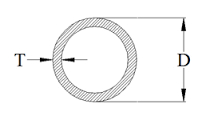Did you find an error or inaccuracy? Feel free to write us. Thank you!Tips to related online calculators
Need help to calculate sum, simplify or multiply fractions? Try our fraction calculator.

## Related math problems and questions:

• Eight 9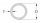Eight pipes are 2¼ meter long . what is the total length of the eight pipes?
• The length 6The length of 12 pipes is 10 1/2 meters. (1) find the length of one pipe (2) also find the length of 7 pipes
• Two ribbonsThe total length of the two ribbons is 13 meters. If one ribbon is 7 and 5/8 meters long, what is the length of the other ribbon?
• Equivalent fractions 2Write the equivalent multiplication expression. 2 1/6÷3/4
• The sum 12The sum of 3 mixed numbers is 20 13/15. two of the numbers are 6 1/3 and 7 5/6. what is the third number?
• Numbers divisionWith what number should be divided mixed number 2 3/4 to get 11/12?
• James 2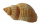James collected 24 seashells on the seashore to be shared with his 2 friends. What fractional part of the seashells will each one get if it is distributed evenly? ​
• Divide 11Divide the product of 4 and 5/8 by 1 1/2. Write your answer as a mixed number.Add this two mixed numbers: 1 5/6 + 2 2/11=
• Cutting pipeA 10⅔ inch long pipe is cut into 8 equal pieces. How long is each piece?
• A triangle 2A triangle has a base of 2 inches and a height of 2 3/4 inches. What is the area of the triangle?
• Between two mixed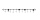What is the rational number between 2 1/4 and 2 4/5?
• Walk for exerciseAnya, Jose, Cali, and Stephan walk for exercise. Anya's route is 2 1/4 kilometers long. Jose's route is 1 1/2 fewer km. Cali's route is 1 1/2  times as long as Jose's route, and 2 fewer km than Stephan's route. What distance (S) is Stephan's route?
• Of the 2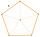Of the shapes,1/6 are triangles and 5/12 are pentagons. What fraction of the shapes are either triangles or pentagons?
• JefferyJeffery is painting a wall the width of the wall is 9 feet the length of the was is 8 2/3 feet what is the area of the wall Jeffery wants to paint?
• Product increased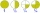When the product of 2/3 and 6/10 is increased by 2/5, the result is?What is 4 1/2+2/7-213/14?